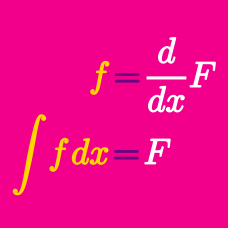Calculus

# Integration of Algebraic Functions

Which function $f(x)$ satisfies $f'(x)=6x^2-8x+9,$ $f(1)=12?$

Suppose $f(x) = 10x + 4$ is a function such that $F'(x) = f(x)$. If the graph of the function $y = F(x)$ passes through the origin, then what is the value of $F(3)$?

Suppose there are functions $F(x)$ and $f(x)$ such that $f(x) = 6x^2 + 6x$ represents the slope of the tangent line to $F(x)$ for all $x$. If the graph of $y = F(x)$ passes through the point (1, 8), then what is the value of $F(3)$?

If constants $a$, $b$, and $c$ satisfy $\frac{d}{dx} \int (ax^2+7x+4)\ dx = 5x^2+bx+c,$ what is $a+b+c$?

If $f(x)=\int \left( 1+2x+3x^2+\cdots + 8x^{7} \right)dx$ and $f(0)=8,$ what is the value of $f(2)?$

×